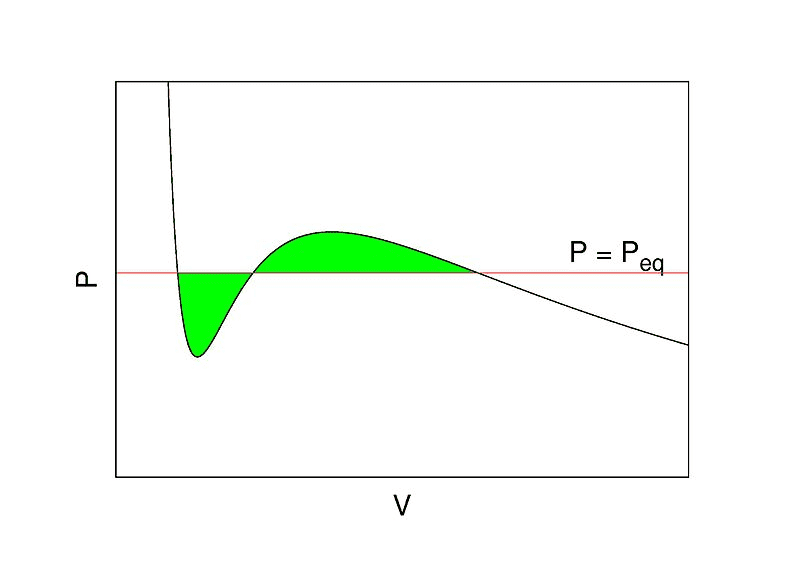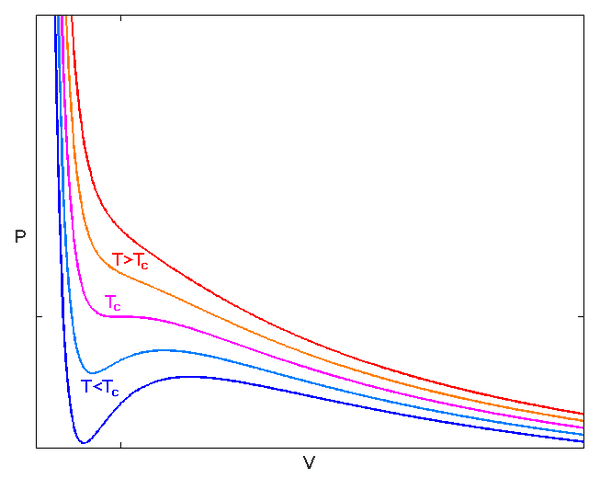# Proof of second-order phase transition for a Van Der Waals Gas

CGandC
Homework Statement:
I was wondering how is it proved that Van Der Waals Gas is a second-order phase transition.
Relevant Equations:
Van Der Waals gas's pressure is: $$P = \frac{NRT}{V-Nb} - \frac{aN^2}{V^2}$$
( This equation does not exist for V<=NB )
How is it proved that Van Der Waals gas is a second order phase transition?
The second order derivative of the pressure (P ) with respect to volume ( V ) don't have a discontinuity ( except at point V = Nb , but the pressure is not existent for V<=Nb ). So how come Van der waals gas describes a second order phase transition?

Homework Helper
Gold Member
## b ## is the volume of each particle, so that ## Nb ## is the smallest volume this collection of particles can occupy. It is unnecessary to look mathematically at cases where ## V \leq Nb ## for the Van der Waals gas.

Gold Member
•CGandC, Lord Jestocost and Charles Link
CGandC
Why do you think this? Can you give a citation?
http://www.pmaweb.caltech.edu/~mcc/Ph127/b/Lecture3.pdf

Actually, after further inquiring about the subject ( I asked a professor teaching statistical physics course ), he told me that the Van-Der-Waals gas is a model characterized by both first order and second order phase transitions, explanation:

The gas describes a first order phase transition because if for example we plot P(v) , we'll get something like this:in the green-shaded area, the following stability-criteria does not hold:

## - \frac{\partial p}{\partial V}>0 ##
( This stability criteria appears in "Thermodynamics and introduction to Thermostatistics - Callen" )
Since the stability-criteria does not hold then we say there is a "jump" of P(v) in the green-shaded area and we have to insert Maxwell-construction model to describe the system's process in the green area.

However, The gas also describes a second order phase transition for a specific type of isotherm - this isotherm describes a temperature called "Critical Temperature" ( also denoted as Tc ), and if we plot P(v) for this isotherm, we'll see that P(v) has stability-criteria fulfilled for all V , but there is a single point in which P(v) has an inflection point - this is the critical point in which the first and second derivatives are zeros ( since this is an inflection point ):
## \frac{\partial p}{\partial V} = \frac{\partial^2 p}{\partial V^2} =0 ##Conclusion: VDW gas desribes mostly a first-order phase transition except for a specific isotherm ( Tc) for which the gas describes only a second order phase transition.

•Gold Member
What if ##T>T_c##?

CGandC
What if ##T>T_c##?

If T>T_c then there is no phase transition since once we have reached T=T_c and went to higher temperature - our VDW model already passed from one phase into another.
So for T>T_c then P(v) describes only one specific phase and no phase transitions since the stability criteria
$$- \frac{\partial p}{\partial V}>0$$
Is satisfied - so there are no discontinuities which would indicate a first-order phase transition and also there are no inflection points which would indicate a second-order phase transition, so P(v) only describes the relationship between P and V for the one particular phase we are currently in for T>T_c.

•TeethWhitener
•